# Neutron 6: 2nd Degree (N6-30)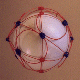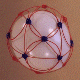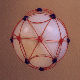A spherical solution for N6. Note: At one time I had a sinusoidal solution off the core structure of N6-30 which had 3 P.V.B.'s, but because it was difficult to form the core structure for this shell, I removed the sinusoidal string and put on fillers to test this structure. The results are what you see. So, there is at least one other solution available off the core structure of this shell.

## Stereograms of N6-30 Shell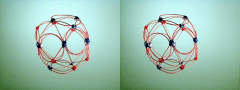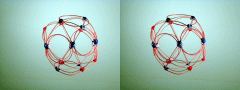Required Snap Points: 66 Available Snap Points: 66 Pinned Vector Bosons: 0 L1 = 29.10494525" (Structural) L2 = 29.10494525" (Structural) L3 = 16.42129106" (Filler) Model Diameter: 9.26439181" Loop Ratio: (1:2): 1 (1:3): 1.772390802 (2:3): 1.772390802 Loop Equation: 3(L1) + 3(L2) + 8(L3) = 306" Snap Point Equation: 3(6) + 3(8) + 8(3) = 66 R.S.P.

# Neutron 6: 3rd Degree, Polar Star (N6-30P)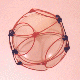This P6 shell locates all 12 Down quarks at the two poles of the shell. It stacks into a complementary shell formation with P6-30PB and P6-40PS. From the typical structures carbon 12 forms, I believe this neutron shell typically takes the lower (inner) shell position with P6-30PB as the outer shell.

## Stereograms of N6-30P Shell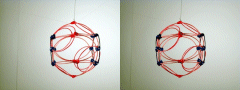Required Snap Points: 66 Available Snap Points: 66 Pinned Vector Bosons: 0 L1 = 27.94145579" (Structural) L2 = 16.84933181" (Filler) L3 = 18.62763719" (Polar) Model Diameter: 10.26995824" Loop Ratios: (1:2): 1.658312395 (1:3): 1.5 (2:3): 0.904534034 Loop Equation: 6(L1) + 6(L2) + 2(L3) = 306" Snap Point Equation: 6(6) + 6(3) + 2(6) = 66 R.S.P.

# Neutron 6: 4th Degree, Polar Star (N6-40P)Similar to the solution above, but with one polar and one equatorial circlet rather than two polar circlets. The down quarks all reside at the equator and the pole opposite the one shown. (Side and opposite pole not shown because I never bothered to actually build the model.) This neutron shell will stack with P5-35P to form a Boron 11 nuclei having all 5 of the proton shells P.V.B.'s clustered at one pole of the P5-35P shell. It will also stack into complementary shells with P6-30PA to form a highly polarized nuclear isomer of carbon 12.

 Required Snap Points: 66 Available Snap Points: 66 Pinned Vector Bosons: 0 L1 = 26.74941211" (Structural) L2 = 30.8875714" (Equatorial) L3 = 16.13050242" (Filler) L4 = 17.83294141" (Polar) Model Diameter: 9.831819336" Loop Ratios: (1:2): 0.8660251 (1:3): 1.658312395 (1:4): 1.5 (2:3): 1.914854887 (2:4): 1.732051415 (3:4): 0.904534034 Loop Equation: 6(L1) + 1(L2) + 6(L3) + 1(L4) = 306" Snap Point Equation: 6(6) + 1(6) + 6(3) + 1(6) = 66 R.S.P.

# Neutron 6: 4th Degree, Belt Sinusoidal (N6-42S)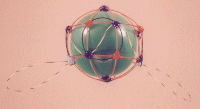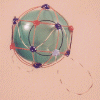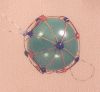Here's an exotic banded solution set for N6 containing an interesting arrangement of strings. The 4 structural loops are equatorial, all intersecting on the down quarks at either pole. A sinusoidal string wraps the equator with pseudo-equatorial circlets making contact at the minima and maxima of the sine. This sinusoidal string is fairly easy to mensurate because the amplitude of the sine can be easily calculated.

## Stereograms of N6-42S ShellRequired Snap Points: 66 Available Snap Points: 64 Pinned Vector Bosons: 2 L1 = 34.78010941" (Structural) L2 = 33.30825351" (Pseudo-Equatorial) L3 = 14.15742257" (Filler) L4 = 43.63336506" (Sinusoidal) Model Diameter: 11.07085267" Loop Ratios: (1:2): 1.044188925 (1:3): 2.456669585 (1:4): 0.797098949 (2:3): 2.352706035 (2:4): 0.7633666 (3:4): 0.32446323 Loop Equation: 4(L1) + 2(L2) + 4(L3) + 1(L4) = 306" Snap Point Equation: 4(6) + 2(8) + 4(4) + 1(8) = 64 A.S.P. P.V.B.'s:(+ 2 P.V.B.'s = 66 R.S.P.)

Copyright 1997 by Arnold J. Barzydlo
Go Back